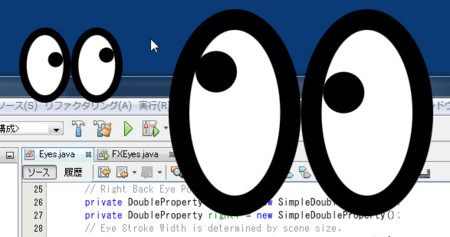# FXEyes

FXEyes is a kind of xeyes. Two eyes follow the mouse cursor movements on the screen.

https://gist.github.com/1523258:titile=FXEyes source

To make FXEyes the location of mouse cursor is needed, but JavaFX doesn't provide a class like MouseInfo class in AWT. Therefore, I used MouseInfo class.
MouseInfo class must work on Swing Event Dispatch Thread (EDT), JavaFX works on JavaFX EDT. Platform.runLater method is used for updating the location of mouse cursor.

Eyes class has properties: size and location et al. These properties value are changed in response to the stage size and location.

```public class Eyes extends Parent {
// Eye Position
private DoubleProperty locationX = new SimpleDoubleProperty();
private DoubleProperty locationY = new SimpleDoubleProperty();
private DoubleProperty width = new SimpleDoubleProperty();
private DoubleProperty height = new SimpleDoubleProperty();

public DoubleProperty locationXProperty() {
return locationX;
}

public DoubleProperty locationYProperty() {
return locationY;
}

public DoubleProperty widthProperty() {
return width;
}

public DoubleProperty heightProperty() {
return height;
}
```

In FXEyes class, these properties are binded to the properties of the stage and the scene.

```        eyes = new Eyes();
eyes.widthProperty().bind(scene.widthProperty());
eyes.heightProperty().bind(scene.heightProperty());
eyes.locationXProperty().bind(stage.xProperty());
eyes.locationYProperty().bind(stage.yProperty());
```

The other property, strokeWidth, is also binded to width and height with some logics. So, strokeWidth is implemented by low-level bind API.

```    private DoubleBinding strokeWidth = new DoubleBinding() {

{
super.bind(width, height);
}

@Override
protected double computeValue() {
// Strok Width is dicided by screen size.
double stroke;
if (width.get() < height.get()) {
stroke = width.get() / SIZE_RATIO;
} else {
stroke = height.get() / SIZE_RATIO;
}
return (stroke < MINIMAM_STROKE) ? MINIMAM_STROKE : stroke;
}
};
```

And then, Eyes class draw ellipse based on the properties.
For example, the center of left eye is (width/4, height/2). To calculate center of eye, divide method of DoubleExpression class is used.

```    private void createEyes() {
// Left Eye
Ellipse left = new Ellipse();
left.centerXProperty().bind(width.divide(4.0));
left.centerYProperty().bind(height.divide(2.0));
left.setStroke(Color.BLACK);
left.strokeWidthProperty().bind(strokeWidth);
left.setFill(Color.WHITE);
```

Next, I'll show udpating black eye positions in response to the location of mouse cursor.

As I have described previously, the location of mouse cursor is get by MouseInfo class. MouseInfo class is worked on Swing EDT and udpating the black eye positions is work on JavaFX EDT. Therefore, I use SwingUtilities.invokeLater method and Platform.runLater method.

```    private void startSwingEDT() {
SwingUtilities.invokeLater(new Runnable() {
@Override
public void run() {
timer = new Timer(50, new ActionListener() {
@Override
public void actionPerformed(java.awt.event.ActionEvent event) {
final PointerInfo info = MouseInfo.getPointerInfo();

Platform.runLater(new Runnable() {
@Override
public void run() {
eyes.setMouseLocation(info.getLocation().getX() - scene.getX(),
info.getLocation().getY() - scene.getY());
}
});
}
});
timer.start();
}
});
}
```

setMouseLocation method shows on below:

```    public void setMouseLocation(double mouseX, double mouseY) {
double x = mouseX - locationX.get();
double y = -mouseY + locationY.get() + height.get();
double cx = width.get() / 4;
double cy = height.get() / 2;

// Update Left Black Eye Position
Point2D left = calculateEyePosition(x, y, cx, cy);
leftX.set(left.getX());
leftY.set(left.getY());

// Update Right Black Eye Position
cx = width.get() / 4 * 3;
Point2D right = calculateEyePosition(x, y, cx, cy);
rightX.set(right.getX());
rightY.set(right.getY());
}

private Point2D calculateEyePosition(double x, double y, double cx, double cy) {
double theta = Math.atan2(y - cy, x - cx);
double hr = width.get() / 4 - 2 * strokeWidth.get();

double eyeX;
double eyeY;

if (Math.abs(x - cx) > Math.abs(hr * Math.cos(theta))) {
eyeX = cx + hr * Math.cos(theta);
} else {
eyeX = x;
}

double vr = height.get() / 2 - 2 * strokeWidth.get();
if (Math.abs(y - cy) > Math.abs(vr * Math.sin(theta))) {
eyeY = height.get() - cy - vr * Math.sin(theta);
} else {
eyeY = height.get() - y;
}

return new Point2D(eyeX, eyeY);
}
```

leftX, leftY, rightX, rightY are properties binded to black eye positions.

```        // Left Black Eye
Circle leftBlackEye = new Circle();
leftBlackEye.centerXProperty().bind(leftX);
leftBlackEye.centerYProperty().bind(leftY);